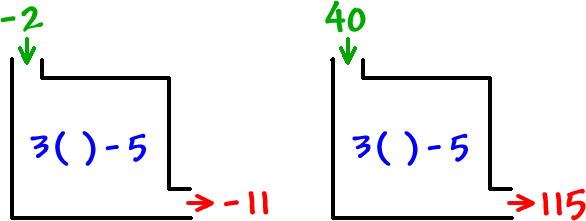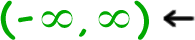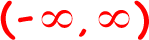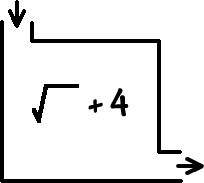One last box:

THE RULE:  Triple the input, then subtract 5What's the domain and range for this function?

domain  =  all real numbers

 =interval notation

 range  =TRY IT:

What's the domain and range for this function?

THE RULE:  Take the square root, then add 4*We're only dealing with real numbers -- no imaginary guys!